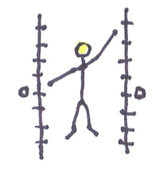Kinesthetics

Kinesthetic Function DiagramsHenri Picciotto

(To understand this, you need to already be familiar with function diagrams.)

I start by doing the "function diagram dance" for my students. The idea of the dance is that my right hand represents x and my left hand represents y. (From the student's perspective, this puts the x on the left and the y on the right, where they should be.) As I move my x hand from negative infinity (all the way down) to positive infinity (all the way up), I move my y hand in various ways, for example exactly along the x, for y=x, or consistently ahead of the x, for y=x+1, or twice as fast as the x for y=2x. Each time, the students have to figure out the function I am representing. (This only works for very simple functions, such as y=mx, or y=x+b.) After dancing some examples myself, I ask the students to do the dance, at first with functions similar to mine, then perhaps some new ones such as for example for y=-x and y=-2x.

I usually close the door right before doing this. This way they don't have to worry about being seen.

:)